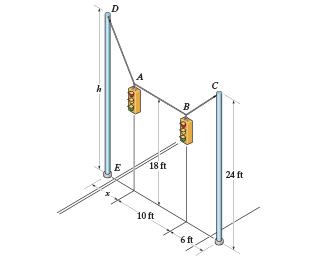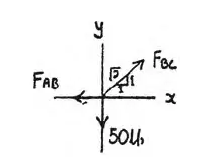×
Get Full Access to Engineering Mechanics: Statics - 14 Edition - Chapter 3 - Problem 3-28
Get Full Access to Engineering Mechanics: Statics - 14 Edition - Chapter 3 - Problem 3-28

×

# Solved: The street-lights at A and B are suspended fromISBN: 9780133918922 126

## Solution for problem 3-28 Chapter 3

Engineering Mechanics: Statics | 14th Edition

• Textbook Solutions
• 2901 Step-by-step solutions solved by professors and subject experts
• Get 24/7 help from StudySoup virtual teaching assistantsEngineering Mechanics: Statics | 14th Edition

4 5 1 308 Reviews
29
4
Problem 3-28

The street-lights at A and B are suspended from the two poles as shown. If each light has a weight of 50 lb, determine the tension in each of the three supporting cables and the required height h of the pole DE so that cable AB is horizontal. D A h B C E 24 ft 18 ft 6 ft 10 ft 5 ft Prob. 328

Step-by-Step Solution:

Problem 3-28

The street-lights at A and B are suspended from the two poles as shown. If each light has a weight of 50 lb, determine the tension in each of the three supporting cables and the required height h of the pole DE so that cable AB is horizontal.Step-by-Step Solution

Step 1 of 2

Use the sum of the forces to solve for all forces in terms of the given angles, then solve for the unknown angle to solve for the height h.

At point B.Step 2 of 2

##### ISBN: 9780133918922

Unlock Textbook Solution